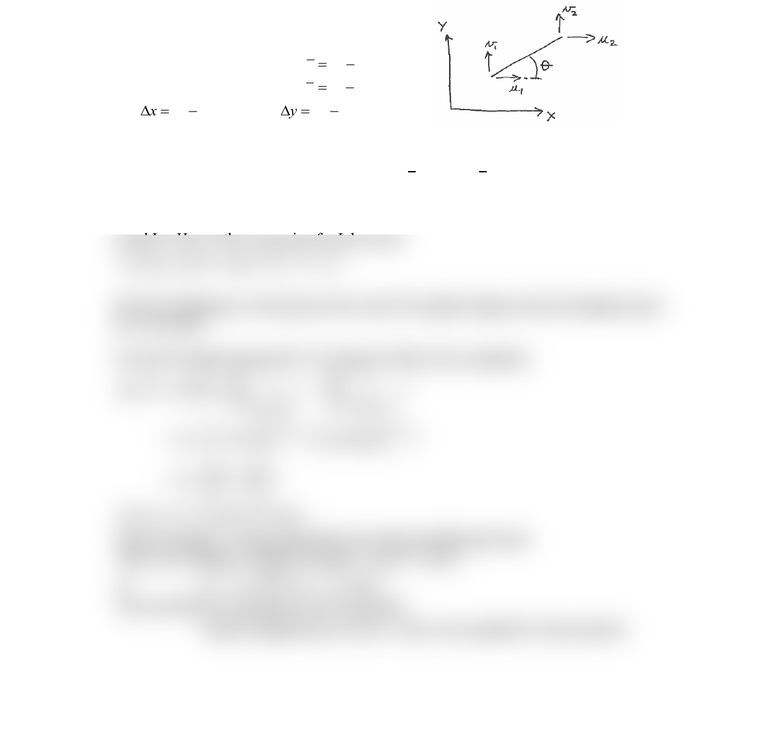Study Guides (390,000)
US (220,000)
TAMU (3,000)
AERO (10)
Final

# AERO 306 Lecture 3: 6_linearApproximationExam

Department
Aerospace Engineering
Course Code
AERO 306
Professor
John Whitcomb
Study Guide
Final

This preview shows half of the first page. to view the full 2 pages of the document.C:\Users\cloudconvert\server\files\119\172\7\5a0dd2d7-a727-436e-b7d3-
aa2efc792964\a1b0b13e51b1a0216306212a529978.docx 06/21/19
Page 1 of 2
Linear Approximation for Two-force Member
This section derives a general linear approximation of the deformation of two-force
members, such as springs and truss members. From earlier classes, you have seen that
even simple truss structures contain a fair number of members. If we do not assume linear
behavior, even simple truss structures would be very difficult to analyze and such
analysis would be well beyond the scope of this class. Fortunately, the linear
approximation is generally quite accurate.
There are various ways to do the derivation. My preferred way is on the next page in
handwritten notes.
Definitions
Displacements at each = u, v
Relative x-displacement =
21
u u u=−
Relative y-displacement =
21
v v v=−
21
x x x =
21
y y y =
Here are expressions for the length of the member before and after deformation.
( ) ( ) ( ) ( )
1/2 1/2
2 2 2 2
0
L x y L x u y v
 
=  + =  + + +
 
If you multiply out the expression for L, you will find some of the terms combine to
equal Lo. Hence, the expression for L becomes
1/ 2
2 2 2
022L L xu y v u v

= + + + +

Now the challenge is to determine how much the length changes when the displacements
are very small.
Let's get an approximation for L by using a Taylor series expansion
1/2 1/2
22
0 0 0
0
00
0
0, 0
0, 0
( , ) (0,0)
11
22
22
( , ) cos sin
where the angle is determined from the initial (undeformed) state
||
uv
uv
LL
L u v L u v
uv
L x L u y L v
xy
L u v
LL
L u v L u v

−−
==
==

= + +

= + +

= + +
= + +
2 1 2 1
That is, the change in length is simply cos sin
or ( ) cos (v v ) sin
This could also be expressed as the dot product
(relative displacement vector) (un
uv
uu


+
+
it vector parallel to truss member)